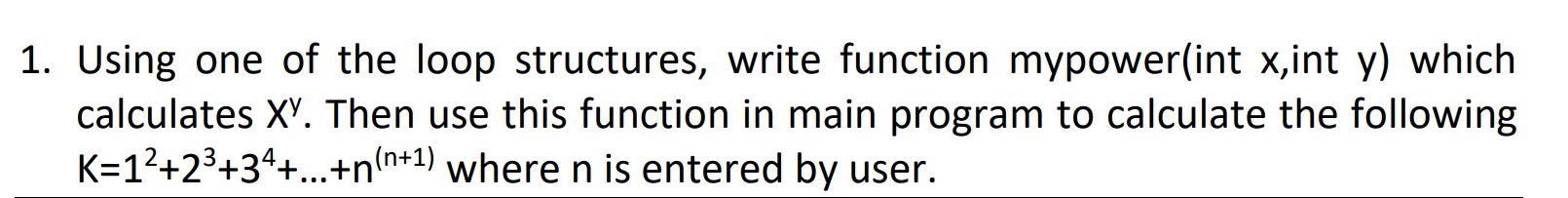# c++ problem ...I have this problem.. this is the code:

#include<iostream>
using namespace std;

int mypower(int x, int y)
{
int Result;

for (int i = x; i <= x; i++);
{
Result = pow(x, y);
return Result;

}

}
int main()
{
int value1, value2;
cout << "Enter a Value Please: ";
cin >> value1 ;
value2 = value1 + 1;

cout << "The result is: " << mypower(value1, value2) << endl;

return 0;
}

What's the problem in the code ?

answered Nov 18 by (12,220 points)

When you compile your code (try to run it) the compiler tells you if there are any errors. In your case, this is the error:

Compilation failed due to following error(s).

```main.cpp: In function ‘int mypower(int, int)’:
main.cpp:12:14: error: ‘pow’ was not declared in this scope
Result = pow(x, y);
^~~```

This means, that the compiler has no idea what `pow` is. Rightfully so, as this function is part of <cmath> library. So all you need to do is include it:

`#include <cmath>`

Furthermore, since you're writing C++ code and not C (in which case you'd do #include <math.h> instead) you should prefix your standard library functions with std::. However, since you included a using namespace std; statement, this is not necessary anymore.

# Unusual code logic

In your function mypower() you have a loop what starts at x and ends when the loop variable (i) grows beyond x. So this loop only runs once. Furthermore, in the body of the loop you return unconditionally, so again, even if the loop conditions wouldn't limit your loop to happen only once, the return statement would force it anyway.

So why do you have a loop there anyway?

Your mypower() function could have been as simple as:

```int mypower(int x, int y)
{
return std::pow(x, y);
}```